Excel Tips & Tutorials:
Hypothesis Tests with One and Two Samples
 Suggest a Link Alphabetize Page Printer-Friendly List
Watch and Learn
Watch and Learn
Watch and Learn
Watch and Learn
 How to Test for Equal and Unequal Variance (F Test)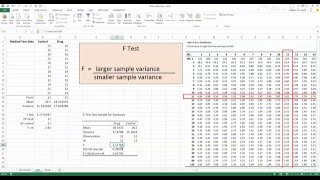Eugene O'Loughlin shows how to use an F test to decide whether to assume equal variances (7:22)Independent Samples T-Test from Only Sample Size, Mean, and Standard Deviation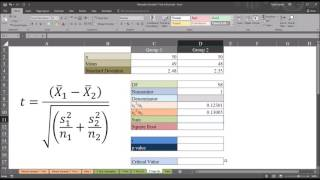Todd Grande shows how to conduct t-test from descriptive statistics (7:38)Testing Difference of Two Proportions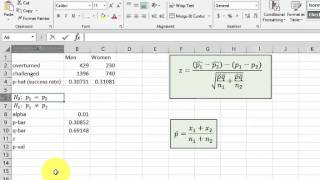Matt Vespa solves a sample problem (5:45).Paired Samples t-Test in Excel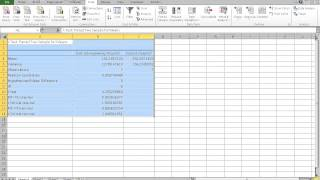From Matt Macarty (9:45)How to Test for Equal and Unequal Variance (F Test)Eugene O'Loughlin shows how to use an F test to decide whether to assume equal variances (7:22)Independent Samples T-Test from Only Sample Size, Mean, and Standard DeviationTodd Grande shows how to conduct t-test from descriptive statistics (7:38)Testing Difference of Two ProportionsMatt Vespa solves a sample problem (5:45).How to Test for Equal and Unequal Variance (F Test)Eugene O'Loughlin shows how to use an F test to decide whether to assume equal variances (7:22)Independent Samples T-Test from Only Sample Size, Mean, and Standard DeviationTodd Grande shows how to conduct t-test from descriptive statistics (7:38)How to Test for Equal and Unequal Variance (F Test)Eugene O'Loughlin shows how to use an F test to decide whether to assume equal variances (7:22)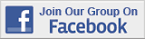Over 18,000 Members!